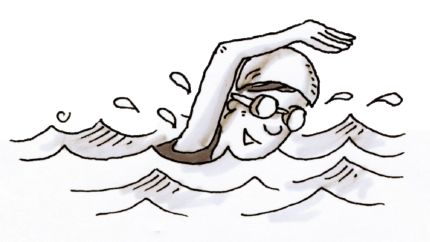### Home > CC2MN > Chapter 9 > Lesson 9.3.2 > Problem9-109

9-109.Michaela holds her state high school record for the $500$-meter freestyle swimming event. She can swim the event in $4$ minutes and $50$ seconds. At this same rate, how far will she swim in $10$ minutes?

Identify this as a proportional relationship problem.

You know that $500$ meters is to $4$ minutes and $50$ seconds.
You will use this to find the distance that would go with $10$ minutes.
Now use one of the three methods of solving proportions to find the answer to this problem.
Convert seconds to minutes to make the calculation easier (It will be a fraction).

Cross multiplication method: $\frac{500\text{ meters}}{4\frac{5}{6}\text{ minutes}}=\frac{x}{10\text{ minutes}}$

$500(10)=x\left(4\frac{5}{6}\right)$   Solve for $x$.

$1034.48$ meters